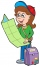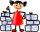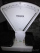# Fraction calculator

The calculator performs basic and advanced operations with fractions, expressions with fractions combined with integers, decimals, and mixed numbers. It also shows detailed step-by-step information about the fraction calculation procedure. Solve problems with two, three, or more fractions and numbers in one expression.

## Result:

### 33/5 x (-81/3) = -30/1 = -30

Spelled result in words is minus thirty.

### How do you solve fractions step by step?

1. Conversion a mixed number 3 3/5 to a improper fraction: 3 3/5 = 3 3/5 = 3 · 5 + 3/5 = 15 + 3/5 = 18/5

To find a new numerator:
a) Multiply the whole number 3 by the denominator 5. Whole number 3 equally 3 * 5/5 = 15/5
b) Add the answer from previous step 15 to the numerator 3. New numerator is 15 + 3 = 18
c) Write a previous answer (new numerator 18) over the denominator 5.

Three and three fifths is eighteen fifths
2. Conversion a mixed number -8 1/3 to a improper fraction: -8 1/3 = -8 1/3 = -8 · 3 + (-1)/3 = -24 + (-1)/3 = -25/3

To find a new numerator:
a) Multiply the whole number -8 by the denominator 3. Whole number -8 equally -8 * 3/3 = -24/3
b) Add the answer from previous step -24 to the numerator 1. New numerator is -24 + 1 = -23
c) Write a previous answer (new numerator -23) over the denominator 3.

Minus eight and one third is minus twenty-three thirds
3. Multiple: 18/5 * (-25/3) = 18 · (-25)/5 · 3 = -450/15 = -30 · 15/1 · 15 = -30
Multiply both numerators and denominators. Result fraction keep to lowest possible denominator GCD(-450, 15) = 15.
In other words - eighteen fifths multiplied by minus twenty-five thirds = minus thirty.

#### Rules for expressions with fractions:

Fractions - use the slash “/” between the numerator and denominator, i.e., for five-hundredths, enter 5/100. If you are using mixed numbers, be sure to leave a single space between the whole and fraction part.
The slash separates the numerator (number above a fraction line) and denominator (number below).

Mixed numerals (mixed fractions or mixed numbers) write as non-zero integer separated by one space and fraction i.e., 1 2/3 (having the same sign). An example of a negative mixed fraction: -5 1/2.
Because slash is both signs for fraction line and division, we recommended use colon (:) as the operator of division fractions i.e., 1/2 : 3.

Decimals (decimal numbers) enter with a decimal point . and they are automatically converted to fractions - i.e. 1.45.

The colon : and slash / is the symbol of division. Can be used to divide mixed numbers 1 2/3 : 4 3/8 or can be used for write complex fractions i.e. 1/2 : 1/3.
An asterisk * or × is the symbol for multiplication.
Plus + is addition, minus sign - is subtraction and ()[] is mathematical parentheses.
The exponentiation/power symbol is ^ - for example: (7/8-4/5)^2 = (7/8-4/5)2

#### Examples:

subtracting fractions: 2/3 - 1/2
multiplying fractions: 7/8 * 3/9
dividing Fractions: 1/2 : 3/4
exponentiation of fraction: 3/5^3
fractional exponents: 16 ^ 1/2
adding fractions and mixed numbers: 8/5 + 6 2/7
dividing integer and fraction: 5 ÷ 1/2
complex fractions: 5/8 : 2 2/3
decimal to fraction: 0.625
Fraction to Decimal: 1/4
Fraction to Percent: 1/8 %
comparing fractions: 1/4 2/3
multiplying a fraction by a whole number: 6 * 3/4
square root of a fraction: sqrt(1/16)
reducing or simplifying the fraction (simplification) - dividing the numerator and denominator of a fraction by the same non-zero number - equivalent fraction: 4/22
expression with brackets: 1/3 * (1/2 - 3 3/8)
compound fraction: 3/4 of 5/7
fractions multiple: 2/3 of 3/5
divide to find the quotient: 3/5 ÷ 2/3

The calculator follows well-known rules for order of operations. The most common mnemonics for remembering this order of operations are:
PEMDAS - Parentheses, Exponents, Multiplication, Division, Addition, Subtraction.
BEDMAS - Brackets, Exponents, Division, Multiplication, Addition, Subtraction
BODMAS - Brackets, Of or Order, Division, Multiplication, Addition, Subtraction.
GEMDAS - Grouping Symbols - brackets (){}, Exponents, Multiplication, Division, Addition, Subtraction.
Be careful, always do multiplication and division before addition and subtraction. Some operators (+ and -) and (* and /) has the same priority and then must evaluate from left to right.

## Fractions in word problems:

• Simplest form of a fractionWhich one of the following fraction after reducing in simplest form is not equal to 3/2? a) 15/20 b) 12/8 c) 27/18 d) 6/4
• Equivalent expressionsA coach took his team out for pizza after their last game. There were 14 players, so they had to sit in smaller groups at different tables. Six players sat at one table and got 4 small pizzas to share equally. The other players sat at the different table
• Math testBrayden was solving some math problems for the math team. He answered 2 math problems. Matthew answered 3, John answered 1 reasoning. Matthew 1/2 times as many. Brayden said that 2/6. Is he correct? Why or why not? Be sure to explain your answer.
• Leo hikedLeo hiked 6/7 of a kilometer. Jericho hiked 2/3 kilometer. Who covered a longer distance? How much longer?
• Colored blocksTucker and his classmates placed colored blocks on a scale during a science lab. The brown block weighed 8.94 pounds, and the red block weighed 1.87 pounds. How much more did the brown block weigh than the red block?
• TroopsThe route is long 147 km and the first-day first regiment went at an average speed of 12 km/h and journey back 21 km/h. The second day went second regiment the same route at an average speed of 22 km/h there and back. Which regiment will take route longer
• DividendsThe three friends divided the win by the invested money. Karlos got three-eighths, John 320 permille, and the rest got Martin. Who got the most and which the least?
• Roma ateRoma ate 2/5 of a cake while Somya ate 3/7 of the same cake. Who ate more and by how much?
• Paper collectingAt the paper collecting contest gathered Franta 2/9 ton, Karel 1/4 ton, and Patrick 19/36 tons of paper. Who has gathered the most and the least?
• Evaluate mixed expressionsWhich of the following is equal to 4 and 2 over 3 divided by 3 and 1 over 2? A. 4 and 2 over 3 times 3 and 2 over 1 B. 14 over 3 times 2 over 7 C. 14 over 3 times 7 over 2 D. 42 over 3 times 2 over 31
• SandySandy, John and Marg baked pies for the Bake Sale. Sandy cut his pies into 6ths, John but his into 8ths, and Marg cut hers into quarters. Sandy sold 11/6, John sold  1 3/8 pies, and Marg sold 9/4 pies. Who sold the most pies? Who sold the fewest?
• Stones in aquariumIn an aquarium with a length 2 m; width 1.5 m and a depth of 2.5 m is a water level up to three-quarters of the depth. Can we place stones with a volume of 2 m3 into the aquarium without water being poured out?
• SayavongSayavong is making cookies for the class. He has a recipe that calls for 3 and 1/2 cups of flour. He has 7/8 of a cup of wheat flour, and 2 and 1/2 cups of white flour. Does Mr. Sayavong have enough flour to make the cookies?